PHY_10_15_V1_DM_The law of universal gravitation
Оценка 4.9

# PHY_10_15_V1_DM_The law of universal gravitation

Оценка 4.9
rtf
02.05.2020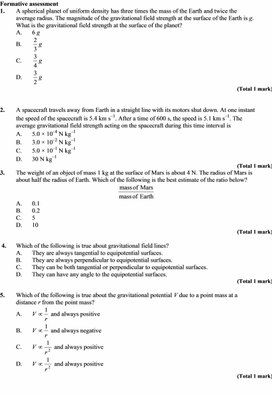PHY_10_15_V1_DM_The law of universal gravitation.rtf

Formative assessment

1.       A spherical planet of uniform density has three times the mass of the Earth and twice the average radius. The magnitude of the gravitational field strength at the surface of the Earth is g.
What is the gravitational field strength at the surface of the planet?

A.      6 g

B.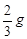C.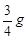D.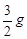(Total 1 mark)

2.       A spacecraft travels away from Earth in a straight line with its motors shut down. At one instant the speed of the spacecraft is 5.4 km s–1. After a time of 600 s, the speed is 5.1 km s–1. The average gravitational field strength acting on the spacecraft during this time interval is

A.      5.0 × 10–4 N kg–1

B.      3.0 × 10–2 N kg–1

C.      5.0 × 10–1 N kg–1

D.      30 N kg–1

(Total 1 mark)

3.       The weight of an object of mass 1 kg at the surface of Mars is about 4 N. The radius of Mars is about half the radius of Earth. Which of the following is the best estimate of the ratio below?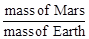A.      0.1

B.      0.2

C.      5

D.      10

(Total 1 mark)

4.      Which of the following is true about gravitational field lines?

A.      They are always tangential to equipotential surfaces.

B.      They are always perpendicular to equipotential surfaces.

C.      They can be both tangential or perpendicular to equipotential surfaces.

D.      They can have any angle to the equipotential surfaces.

(Total 1 mark)

5.       Which of the following is true about the gravitational potential V due to a point mass at a distance r from the point mass?

A.      V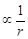and always positive

B.      Vand always negative

C.      V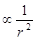and always positive

D.      Vand always positive

(Total 1 mark)

Free response questions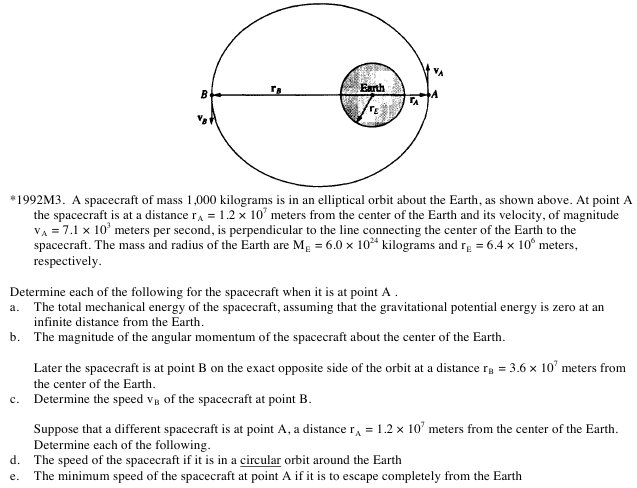Additional problem for differentiation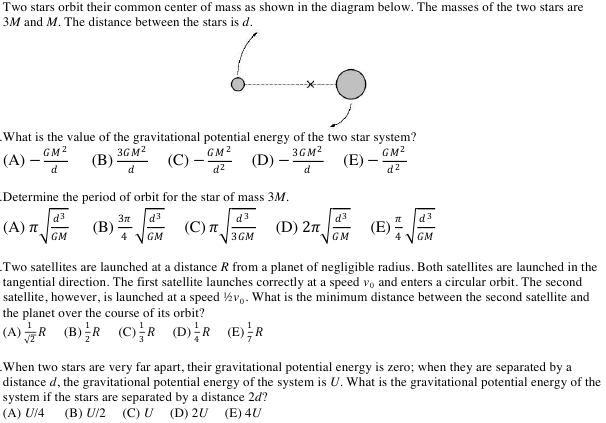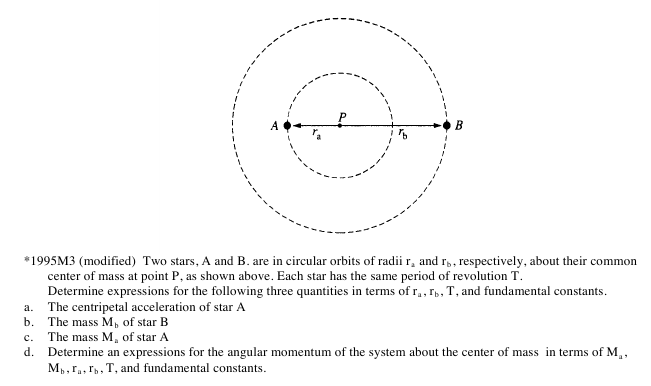Mark scheme of FA

1.       C



2.       C



3.       A



4.       B



5.       B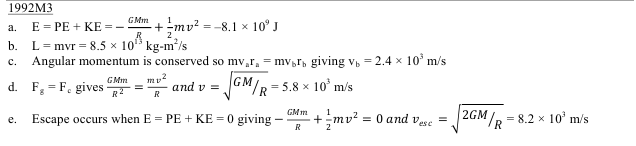Answers to additional problem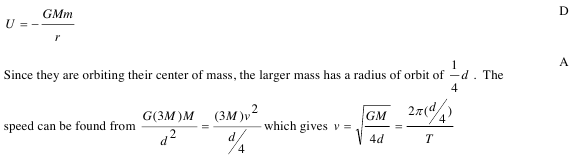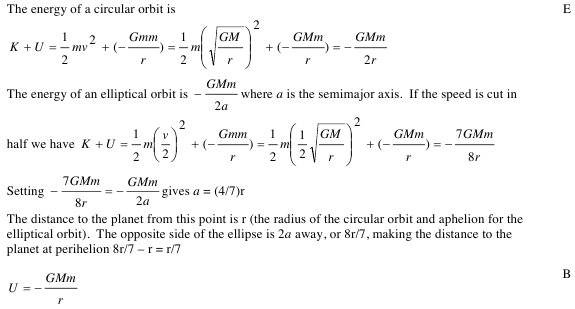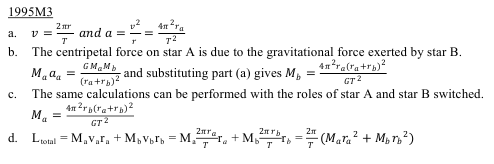Скачать файл